# Column-I Column- II (A) Two vertices of a triangle areand. If orthocentre of the third vertex are (p)(B) A point on the line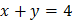which lies at a unit distance from the lineis (q)(C) Orthocentre of the triangle formed by the linesis (r)(D) Ifare in A.P., then linesare concurrent at (s)CODES :   A B C D     a) P q s r     b) t r s p     c) q p r t     d) s p q r

## Question ID - 55399 :- Column-I Column- II (A) Two vertices of a triangle areand. If orthocentre of the third vertex are (p)(B) A point on the linewhich lies at a unit distance from the lineis (q)(C) Orthocentre of the triangle formed by the linesis (r)(D) Ifare in A.P., then linesare concurrent at (s)CODES :   A B C D     a) P q s r     b) t r s p     c) q p r t     d) s p q r

3537

(a)

1.(i)(ii)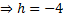Hence, point,is1.(i)(ii)

Letbe the point on (i). Then,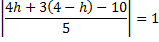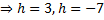Hence, the required point is either (3, 1) or2. Since linesandare perpendicular, orthocentre of the triangle is the point of intersection of these lines, i.e,3. Since,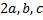are in A.P., soComparing with the line, we haveand. Hence, lines are concurrent atNext Question :
 Column-I Column- II (A) If linesandare concurrent, then value ofis (p)(B) If the pointsandare collinear, then the value ofis (q)(C) If line, passing through the intersection ofand, is perpendicular to one of them, then the value ofis (r) 4 (D) If lineis equidistant from the pointsand (3, 4) thenis (s) 2 CODES : A B C D a) P,q q s,t r b) s,p q,r t q c) s t,s q p,q d) p,s q,s p,r s